# 1st PUC Statistics Previous Year Question Paper March 2019 (North)

Students can Download 1st PUC Statistics Previous Year Question Paper March 2019 (North), Karnataka 1st PUC Statistics Model Question Papers with Answers helps you to revise the complete Karnataka State Board Syllabus and score more marks in your examinations.

## Karnataka 1st PUC Statistics Previous Year Question Paper March 2019 (North)

Time: 3 Hrs 15 Min
Max. Marks: 100

Note :

1. Graph sheets and statistical tables will be supplied on request
2. Scientific calculators are allowed
3. All working steps should be clearly shown.

Section-A

I. Answer any ten of the following questions: (10 × 1 = 10)

Question 1.
Define statistics according to Croxton and Cowden’s.
Statistics is the science of collection, presentation, analysis and interpretation of numerical data.

Question 2.
Define population.
The total number of units under consideration is called population.

Question 3.
What is sampling?
It is method of collection of the data based on a part or a portion of representative items from the population.

Question 4.
Mention One objective of classification.
Classification reduces the size of the data.Question 5.
Write the formula to find the mid-point of a class.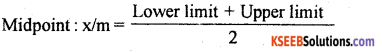Question 6.
Which graph is used to locate median.
Ogives are used to locate median.

Question 7.
Find the geometric mean of 3 and 27.
G.M = $$\sqrt{3 \times 27}=\sqrt{81}=\sqrt{9^{2}}$$ = 9

Question 8.
Define Kurtosis.
Kurtosis means peakedness or steepness of a frequency curve as compared to a normal curve.

Question 9.
For data, if M = 25 then what is value of P50?
P50 = 25

Question 10.
Give the range of correlation co-efficient.
γ = ± 1 or – 1 ≤ γ ≤ + 1

Question 11.
What is interpolation?
Interpolation is the procedure of estimating the unknown value of the dependent variable for a given value of the independent variable which is within the limits of independent variable.

Question 12.
If P (A) = $$\frac { 1 }{ 4 }$$ then find P (A1).
P(A1) = l – P(A) = l – $$\frac { 1 }{ 4 }$$ = $$\frac { 3 }{ 4 }$$

Section-B

II. Answer any ten of the following questions: (10 × 2 = 20)

Question 13.
Write the limitations of statistics.

1. Statistics does not deal with qualitative data. It deals with only quantitative data.
2. Statistical results are riot exact.

Question 14.
What is primary data? Mention one method of collection of primary data.
Primary data are those which are directly collected by the investigator himself initialls for his own purpose.
Method of collection of primary date is direct personal interview method.

Question 15.
Mention two parts of a table.
The parts of table areQuestion 16.
Define range and class limits.
Range is the difference between the highest and the lowest value in the data.
The lowest and the highest values which are taken to define the boundaries of a class are called class limits.

Question 17.
Mention two uses of diagrams and graphs.

1. They give a birds eye view of the entire data at a glance.
2. They can be easily understood by the common people.

Question 18.
If n1 = 100, n2 = 150, X̄1 = 10.4 and X̄2 = 10.9 Find the combined mean.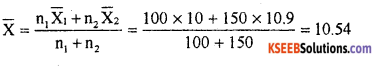Question 19.
For a data, if median is 45 and the mean deviation from median is 10 then find its co¬. efficients.
Co – efficient of mean deviation :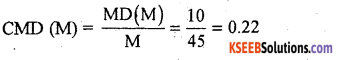Question 20.
If bxy = $$\frac { -3 }{ 4 }$$ and byx = $$\frac { -1 }{ 3 }$$ find the correlation co-efficient
γ = ± $$\sqrt{\mathrm{bxy}, \mathrm{byx}}$$
γ = $$\sqrt{\frac{-3}{4} \times \frac{-1}{3}}$$ = ± 0.5

Question 21.
Give any two properties of regression lines.

1. Two regression lines coincide at (x̄, ȳ)
2. Two regression lines intersect when there is a perfect correlation.

Question 22.
In case of two attributes, if N = 100, (AB) = 20, (A) = 56 and (B) = 22, then find the remaining classes and their frequencies.
The 2 × 2 contingency table is as shown below.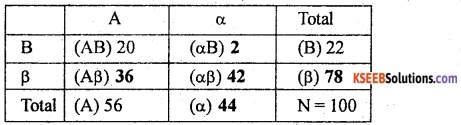Question 23.
Define sample space and equally likely events.
The set of all possible outcomes of a random experiment is called sample space.
Two or more events are said to be equally likely events if they have equal chances of occurrence.

Question 24.
If E(x2) = 74 and E(x) = 5 find the standard deviation.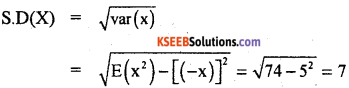Section-C

III. Answer any eight of the following questions . (8 × 5 = 40)

Question 25.
Write a few important functions of statistics.
The following are the important functions of statistics:
(a) It simplifies and condenses the size of the data.
(b) It facilitates composition.
(c) It enriches our knowledge and widens our experience.
(d) It helps in formulating policies.
(e) It helps in business forecasting.
(f) It presents the facts in a definite form.

Question 26.
Mention the five rules for drafting a questionnaire.
Following are some important points/rules to be considered while framing a questionnaire.

1. Questions should be simple and easy to understand and get spontaneous answers.
2. Questions should not confuse the reader and they should give only one meaning.
3. The number of questions should be kept to a minimum.
4. Questions should be such that people with little intelligence and educated ones can both answer without much difficults.
5. The questions should be arranged in a logical order.

Question 27.
Prepare a blank table to show the distribution of students of a College according to
(i) Faculty : Arts, Commerce and Science
(ii) Sex : Male and Female
(iii) Year : 2009 and 2010
Blank table showing the distribution of the college students according to faculty, sex and the year.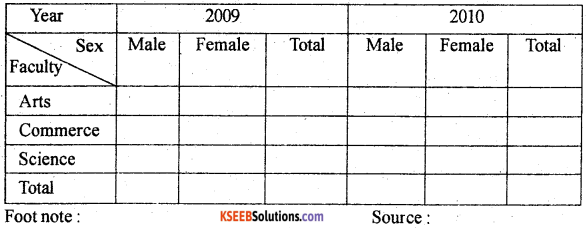Question 28.
Following are the marks obtained by two students A and B in an annual examination. Represent the data by percentage bar diagram.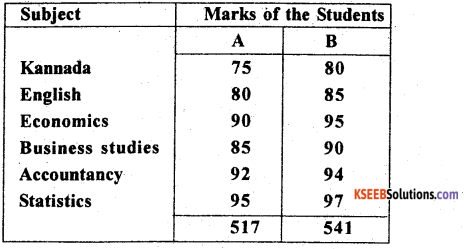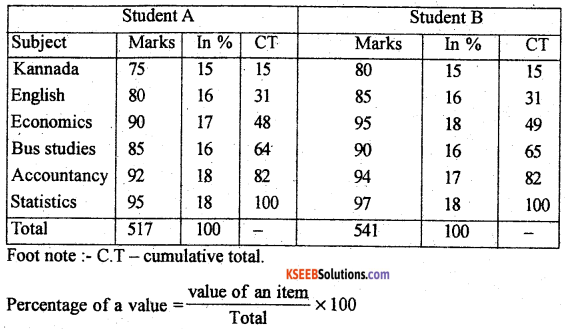e.g.Kannada = $$\frac { 75 }{ 517 }$$ × 100 = 15 %
Percentage bar diagram showing the marks of students A and B.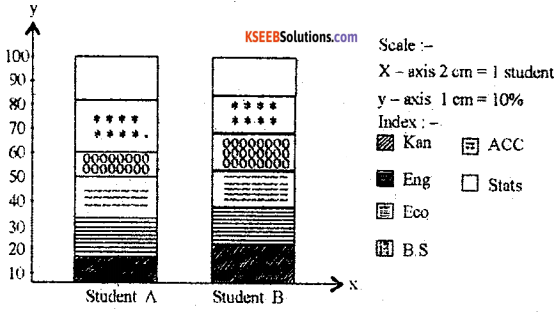Question 29.
Calculate geometric mean from the following data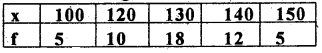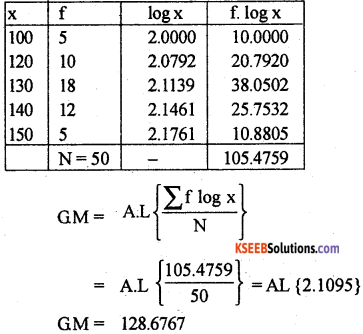Question 30.
Calculate the Spearman’s rank correlation co-efficient for the following data marks of 8 students in statistics (x) and mathematics (y).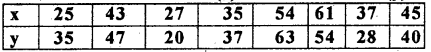Let Rx and Ry be the ranks of X and Y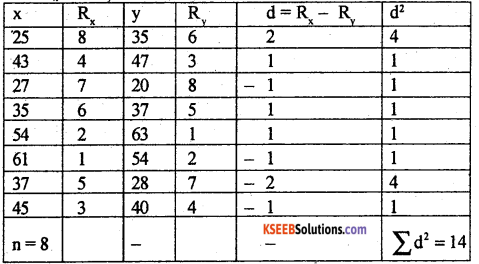Spearman’s rank correlation coefficients: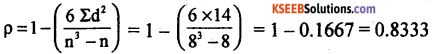There exists a high degree of positive correlation between x and y.

Question 31.
Calculate yule’s association from the following data.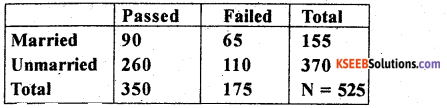The given data can be written under 2 × 2 contingency table as below.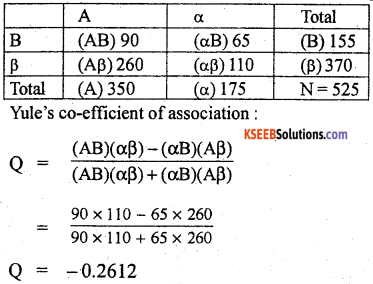There exists a low degree of negative association between marriage and failure.Question 32.
The number of births taking place annually in a village are as follows: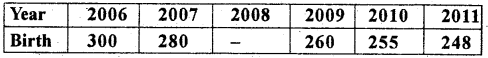Find the missing value for the year 2008.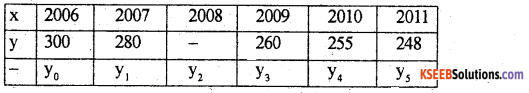Since there are 5 known values of y, so expand the binomial expansion (y – 1)5 = 0,
y55y4 + 10y310y2 + 5y1 – y0 = 0
248 – 5 × 255 + 10 × 260 – 10y2 + 5 × 280 – 300 = 0.
248 – 1275 + 2600 – 10y2 + 1400 – 300 = 0.
y2 = 263.7

Question 33.
State and prove addition theorem of probability for two non-mutually exclusive events.
Statement: Let A and B be any two events (Subsets of sample spaces) with respective probability P(A) and P(B). Then, the probability of occurence of atleast one of these events is:
P(A ∪ B) = P(A) + P(B) – P(A ∩ B)
where P(A ∩ B) is the simultaneous occurence of A and B.

Proof: A random experiment results in ‘n’ exhaustive outcomes out of which movt comes are favourable to event ‘A’, m2 outcomes are favourable to event ‘B’ and ‘p’ outcomes are common to both A and B.
Consider the venn diagram: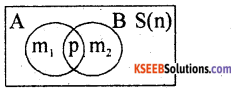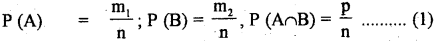The event of occurrence of at least one out of A and B is (A ∪ B) has (m1 + m2 – p) favourable outcomes.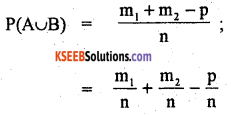Substituting the values from (1)
P(A ∪ B) = P(A) + P(B) – P(A ∩B)
Q.E.D.

Question 34.
A bag contains S white and 4 green balls. Two balls are drawn from the bag at random. Find the probability that they are:
1. of the same colour.
2. of different colours.
n = Two balls can be drawn from 5 white and 4 green balls in 9C2 = 36 ways.
1. m = 2 balls of the same colour can be drawn in (2 W or 2 G) = (5C2 × 4C2) = (10 + 6) = 16 ways.
Let P(A) = P( 2 balls of the same colour) = $$\frac { m }{ n }$$ = $$\frac { 16 }{ 36 }$$ =$$\frac { 4 }{ 9 }$$

2. P(A1) = P(2 balls of different colours) = 1 P(A) = 1 – $$\frac { 4 }{ 9 }$$ = $$\frac { 5 }{ 9 }$$Question 35.
Calculate the Karl Pearson’s co-efficient of correlation from the following data.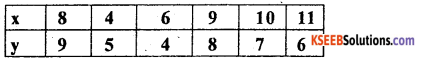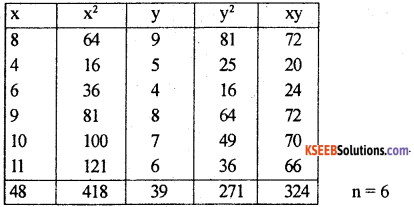Karl Pearson co-efficient of correlation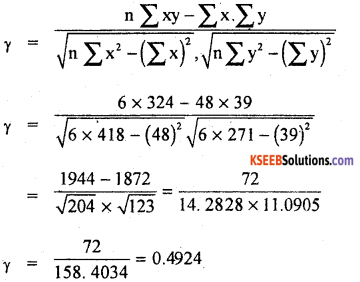There exists a low degree of positive correlation between x and y.

Question 36.
Calculate D5 and P28 from the following data.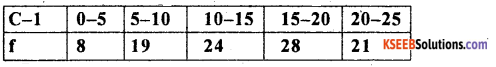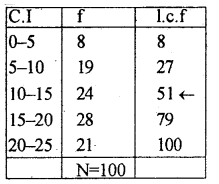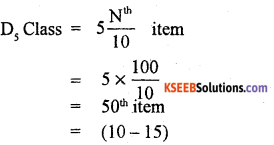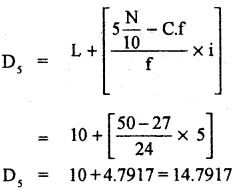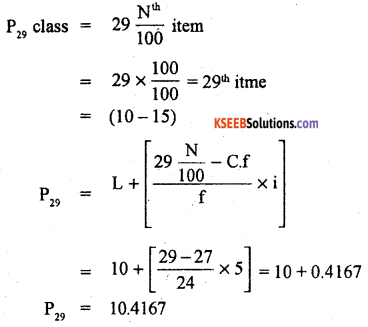Section-D

IV. Answer any two of the following questions: (2 × 10 = 20)

Question 37.
Calculate Mean, Median and Mode from the following data.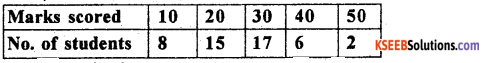Let x and f be the marks and number of students.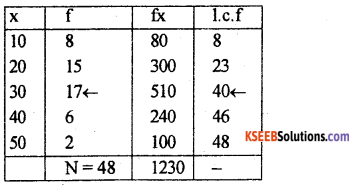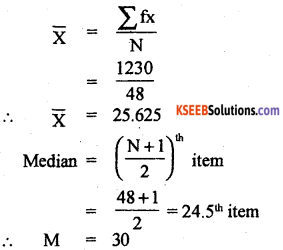Mode = Size of the highest frequency
= Highest frequency is 17. Its size (x) is = 30
∴ z = 30

Question 38.
Calculate Bowley’s co-efficient of Skewness of the following data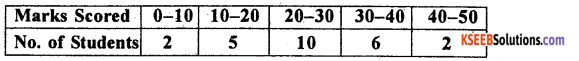Let CI and f be the marks and number of students.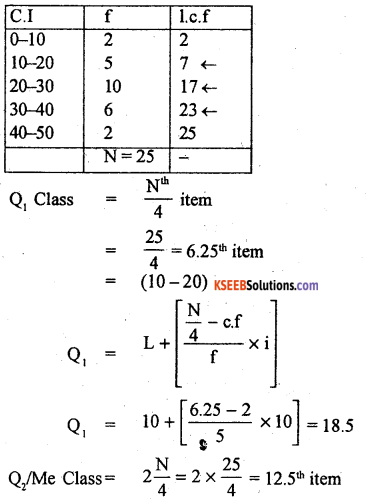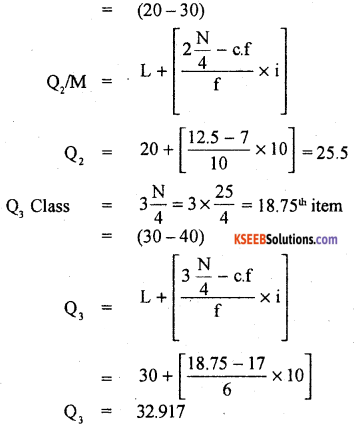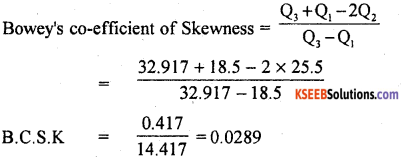There exists a low degree skewness.Question 39.
From the following data find two regression equations.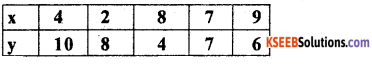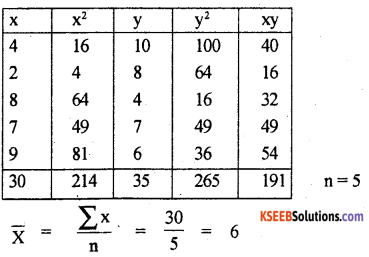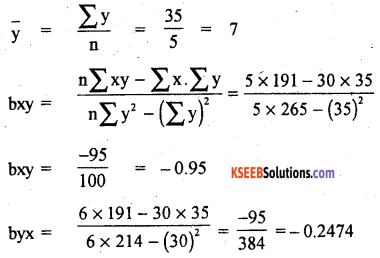Regression equation of x on y:
(x – x̄) = bxy (y – ȳ)
x – 6 = -0. 95 (y -7)
= -0.95 y + 6.65
x = -0.95 y + 6.65 + 6
x = -0.95y + 12.65

Regression equation of y on x:
(y – ȳ) = byx  (x – x̄)
y – 6 = -0.2474 (x – 6)
= -0.2474 x + 1.4844
y = – 0.2474 x + 1.4844 + 6
y = – 0.2474 x + 7.4844.

Question 40.
(a) The probability of two students A and B solving a problem are $$\frac { 1 }{ 2 }$$ and $$\frac { 3 }{ 4 }$$ respectively. If both of them independently try, what is the probability that the problem gets solved.
(b) Find K and mean [E(x)] of the following distribution.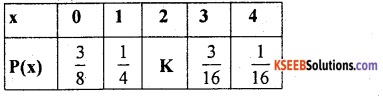(a) Let the probability of student A and B be solving a problem be P (A) = $$\frac { 1 }{ 2 }$$ and P (B) = $$\frac { 3 }{ 4 }$$ Probability that the problem is solved is :-
Probability that at least one will solve is
P(A ∪ B) = P (A) + P (B) – P(A ∩ B)
= P(A) + P(B) – P(A) P(B)
$$=\frac{1}{2}+\frac{3}{4}-\frac{1}{2} \times \frac{3}{4}$$
P(A ∪ B) = 0.5 + 0.75 – 0.375
= 0.875

(b) We know that ΣP(x) = 1
$$\frac{3}{8}+\frac{1}{4}+k+\frac{3}{16}+\frac{1}{16}=1$$
k = 1 – $$\left(\frac{3}{8}+\frac{1}{4}+\frac{3}{16}+\frac{1}{16}\right)$$
= 1 – 0.875
k = 0.125
The probability distribution is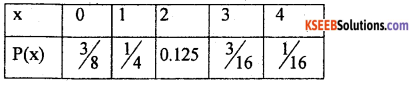Mean = E(x) = Σx P(x)
$$=0 \times \frac{3}{8}+1 \times \frac{1}{4}+2 \times 0.125+3 \times \frac{3}{16}+4 \times \frac{1}{16}$$
E(x) = 1.3125Section-E

V. Answer any two of the following questions : (2 x 5 = 10)

Question 41.
The following are the heights in centimeters of 40 students: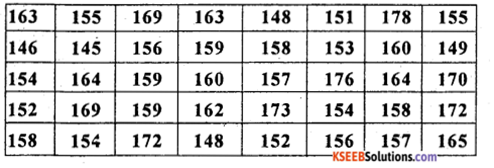From a frequency distribution by exclusive Cl taking lowest class interval as (145 -150) cms
Frequency distribution of height (in cms)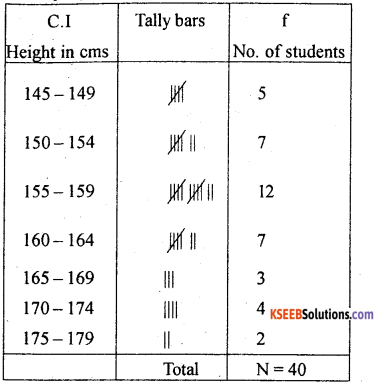Question 42.
Draw a histogram and find the value of mode.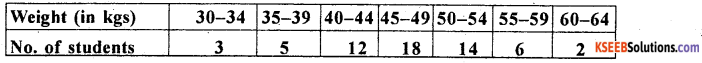Convert inclusive class internal into exclusive class internal.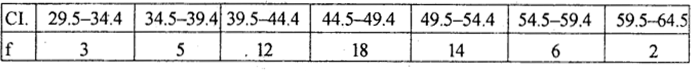Histogram showing the weight of students (in kg)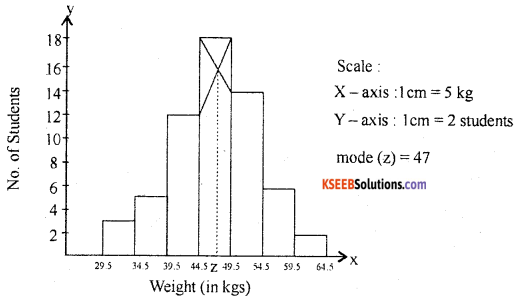Question 43.
The first four central moments of frequency distribution are 0,4,-2 and 2.4. comment on the skewness and Kurtosis.
Given: µ1 = 0, µ2 = 4, µ3 = -2, µ4 = 2.4,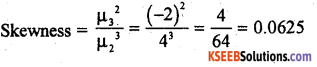The distribution is low degree positive.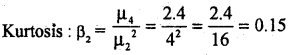Here β2< 3. The distribution platykurtic.

Question 44.
A bag contains 4 white and 5 red marbles. Two marbles are drawn one after the 1 other without changing. Find the probability of getting both as white marbles.
$$=\frac{4}{9} \times \frac{3}{8}=\frac{12}{72}=\frac{1}{6}$$